Courses

# RD Sharma Solutions - Ex-4.1, Rational Numbers, Class 7, Math Class 7 Notes | EduRev

## Class 7: RD Sharma Solutions - Ex-4.1, Rational Numbers, Class 7, Math Class 7 Notes | EduRev

The document RD Sharma Solutions - Ex-4.1, Rational Numbers, Class 7, Math Class 7 Notes | EduRev is a part of the Class 7 Course RD Sharma Solutions for Class 7 Mathematics.
All you need of Class 7 at this link: Class 7

Q 1. Write down the numerator of each of the following rational numbers :

(i) −7/5

(ii) 15/−4

(iii) −17/−21

(iv) 8/9

(v) 5

SOLUTION :

Numerators are :

(i) -7

(ii) 15

(iii) -17

(iv) 8

(v) 5

Q 2 . Write down the denominator of each of the following rational numbers:

(i) −4/5

(ii) 11/−34

(iii) −15/−82

(iv) 15

(v) 0

SOLUTION :

Denominators are :

(i) 5

(ii)  -34

(iii) -82

(iv) 1

(v) 1

Q 3. Write down the rational number whose numerator is (-3) ×4 , and whose denominator is (34 – 23) ×(7 – 4).

SOLUTION :

According to the question :

Numerator = (-3) x 4 = -12

Denominator = (34 – 23) x (7 – 4) = 11 x 3 = 33

Therefore , Rational number = −12/33

Q 4. Write the following rational numbers as integers :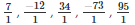SOLUTION :

Integers are 7 , -12 , 34 , -73 and 95 .

Q 5 .Write the following integers as rational numbers with denominator 1 :

-15 , 17 , 85 , -100

SOLUTION :

Rational numbers of given integers with denominator 1 are :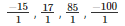Q 6 .Write down the rational whose numerator is the smallest three digit number and denominator is the largest four digit number .

SOLUTION :

Smallest three digit number = 100

Largest four digit number = 9999

Therefore rational number = 100/9999

Q 7. Seperate positive and negative rational numbers from the following rational numbers :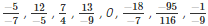SOLUTION :

Given rational numbers can be rewritten as :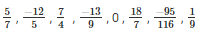Thus , positive rational numbers are :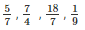Negative rational numbers are :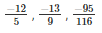Q 8 .Which of the following rational numbers are positive :

(i) −8/7

(ii)  9/8

(iii)  −19/−13

(iv)  −21/13

SOLUTION :

The numbers can be rewritten as :

(i)  −8/7

(ii) 9/8

(iii)  19/13

(iv)  −21/13

The numbers can be rewritten as :Q 9 .Which of the following rational numbers are negative ?

(i)  −3/7

(ii)  −5/−8

(iii)  9/−83

(iv)  −115/−197

SOLUTION :

The numbers can be rewritten as :

(i)  −3/7

(ii)  5/8

(iii)  −9/83

(iv)  115/197

Negative rational numbers are (i) and (iii) .

The document RD Sharma Solutions - Ex-4.1, Rational Numbers, Class 7, Math Class 7 Notes | EduRev is a part of the Class 7 Course RD Sharma Solutions for Class 7 Mathematics.
All you need of Class 7 at this link: Class 7Use Code STAYHOME200 and get INR 200 additional OFF Use Coupon Code
All Tests, Videos & Notes of Class 7: Class 7

### Top Courses for Class 7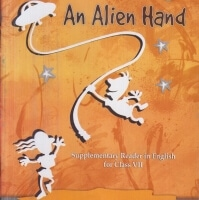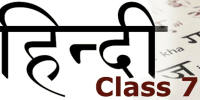## RD Sharma Solutions for Class 7 Mathematics

97 docs

### Top Courses for Class 7Track your progress, build streaks, highlight & save important lessons and more!

,

,

,

,

,

,

,

,

,

,

,

,

,

,

,

,

,

,

,

,

,

,

,

,

,

,

,

,

,

,

;